# Cubes and Dice UPSC Questions

## Cubes and Dice MCQ Questions

1.

A cube has six numbers marked 1, 2, 3, 4, 5 and 6 on its faces. Three views of the cube are shown below: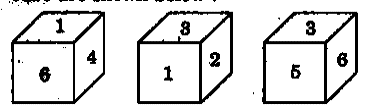What possible numbers can exist on the two faces marked (A) and (B) , respectively on the cube?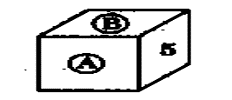UPSC - 2013

A.

2 and 3

B.

6 and 1

C.

1 and 4

D.

3 and 1

A. 2 and 3

2.

Directions for the following 3 questions given below
Rotated positions of a single solid are shown below. The various faces of the solid are marked with different symbols like dots, cross and line. Answer the three items that follow the given figures. What is the symbol on the face opposite to that containing a single dot?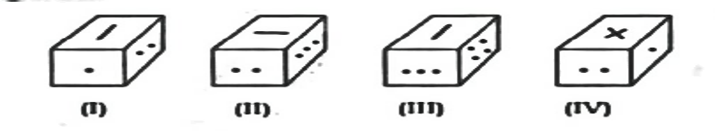UPSC - 2018

A.

Four dots

B.

Three dots

C.

Two dots

D.

Cross

B. Three dots

3.

What is the symbol on the face opposite to that containing two dots?

UPSC - 2018

A.

Single dot

B.

Three dots

C.

Four dots

D.

Line

C. Four dots

4.

What is the symbol on the face opposite to that containing the cross?

UPSC - 2018

A.

Single dot

B.

Two dots

C.

Line

D.

Four dots

C. Line

5.

Six squares are coloured, front and back red(R ), blue(B) , Yellow(Y), green(G ), White(W ) and orange(O) and are binged together as shown in the figure given below. If they are folded to form a cube, what would be the face opposite the white face?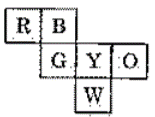UPSC - 2012

A.

Red

B.

Green

C.

Blue

D.

Orange

C. Blue

6.

Three views of a cube following a particular motion are given below: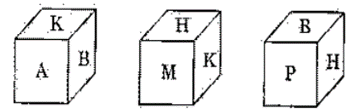What is the letter opposite to A?

UPSC - 2012

A.

H

B.

P

C.

B

D.

M